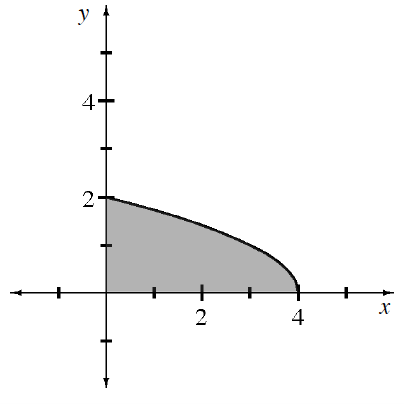### Home > APCALC > Chapter 8 > Lesson 8.3.3 > Problem8-127

8-127.

Multiple Choice: The base of a solid is a region in the first quadrant under the curve $f ( x ) = \sqrt { 4 - x }$, as shown in the diagram at right. The cross-sections of the solid are squares perpendicular to the $x$-axis. What is the volume of the solid?

1. $8$

1. $\frac { 16 } { 3 }$

1. $\frac { 64 } { 9 }$

1. $8π$

1. $\frac { 16 \pi } { 3 }$$\text{volume by cross-sections }=\int_{x=a}^{x=b}(\text{area function})dx$